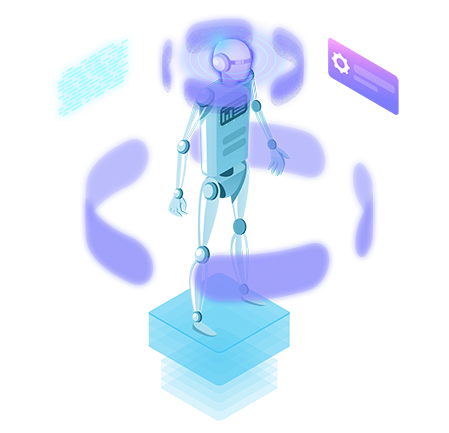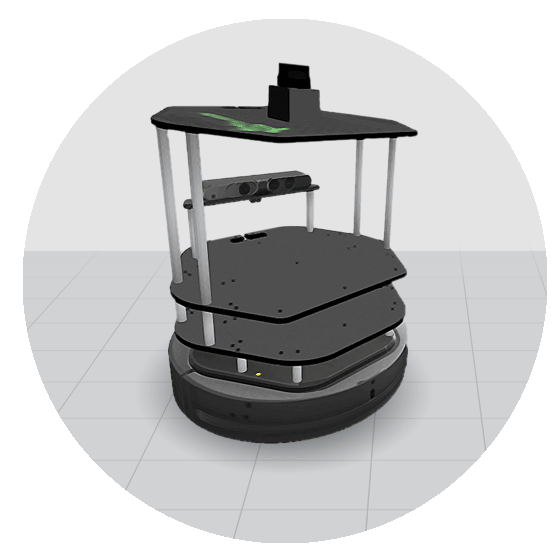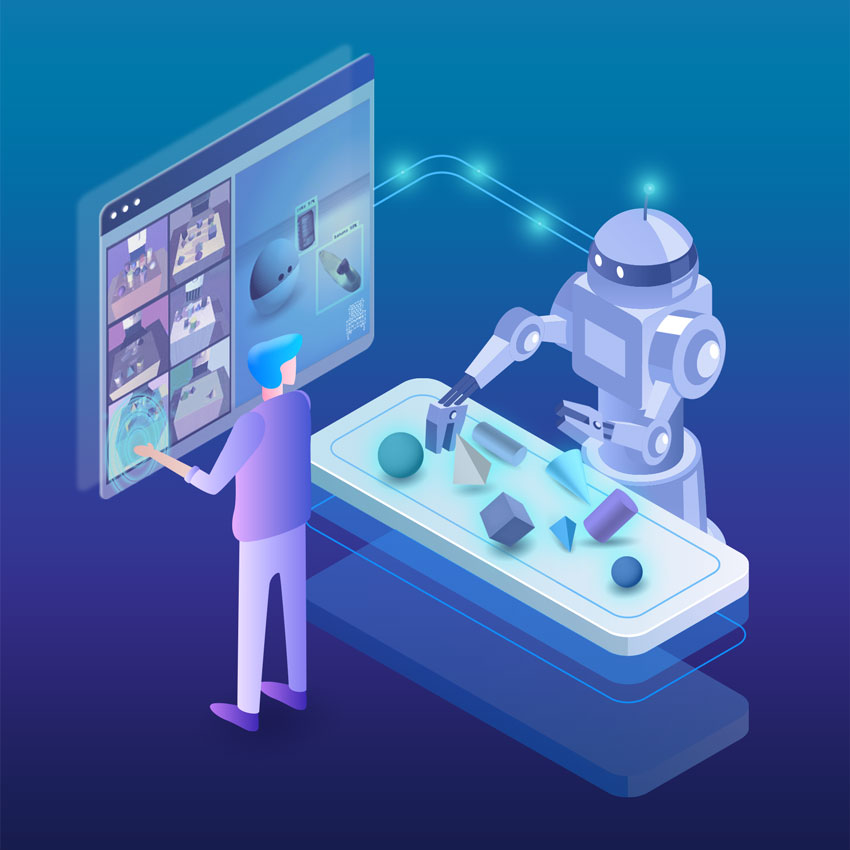# Machine Learning for Robotics Course - Python

Learn about machine learning concepts applicable to robotics; and understand the fundamental principles of artificial intelligence.## Course Summary

In this course, you are going to learn about machine learning concepts applicable to robotics; understand the fundamental principles of artificial intelligence and how the robots think and make independent decisions; make robots smarter and more collaborative; and advance your skills in Python programming.

## What you will learn

• Unit 2: Linear regression (one and multiple variables), logistic regression, and regularization.
• Unit 3: Supervised learning: neural networks, learning using backpropagation.
• Unit 4: Unsupervised learning: k-means algorithm.
• Unit 5: Principal Components Analysis for dimensionality reduction.
• Unit 6: Course PROJECT.

## Course Overview

### Introduction to the Course

Unit for previewing the contents of the Course.

### Linear and logistic regression and regularization

Learn the main concepts of the regression algorithm.

### Supervised learning: neural networks learning using backpropagation

This unit will focus on Neural Networks (NN). We will learn the main concepts of NN: Intuitive understanding of NN (comparison to the brain), the NN concept and main block, Backpropagation algorithm (how to train NN), and how to deploy the NN model in ROS and run applications.

### Unsupervised learning: k-means algorithm

K-mean algorithm approach and implementation. The unit will discuss the K-means algorithm, which you are going to deploy in our robotics ROS domain.

### Principal Components Analysis for dimensionality reduction

In this unit, you are going to continue the discussion regarding unsupervised learning. You will focus on PCA – Principal Component Analysis. This technique is a method of obtaining important variables (in the form of components) from a large set of features available in a data set (you capture a lot of data sets, which are difficult to visualize and make correct decisions).

### Course Project

Your task is to prepare a control application of intelligent radar based on the neural network (NN). The radar is mounted on the TurleBot robot and based on its status, the main robot motion program decides the movement of the robot. The robot moves inside the circuit environment. You should design the radar control system (NN) in such a way that the robot automatically passes the circuit. Normally, there is no limit for the number of circuits the robot passes!

## Teachers

### Markus Buchholz

PhD in Robotics and M.Sc in Electronics and Computer Science and M.sc in Economics. His main passion is programming (C++, Python) the Autonomous Systems by use of AI, Deep Learning and Reinforcement Learning.## Robots used

### Turtlebot robot## Learning Path

Machine Learning for Robots